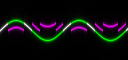Section 2.3 - Min, Max, Mod, Abs and Neg

I will introduce five new functions in this section: min(a,b), max(a,b), a%b, abs(a) and neg(a)

min(a,b): this outputs the smallest value between a and b.

max(a,b): outputs the biggest one.

a%b: a modulo b (remainder of the division of a by b). You will usually have b constant.

abs(a) is |a|, the absolute value of a, ie the same number when it is positive, and the opposite when it's negative...

neg(a) is -a. (But faster than it, as -a is interpreted as 0-a by picFX...)

The min/max functions can be used to fix a bound to some function, having one of the two parameters constant.
Try the following, applying a lowest bound to a sine curve (so we take the *largest* value between the bound and the sine!!)

 (y-128)+max(sin(x/4)*4,-1)(In the picture, I put as green component the same formula without the max, to better view the bound..)

But using two different functions for the arguments is very common as well. For instance if you built separately several parts of a picture and want to put them together, you'll usually use the max() function for that...
You'll see an example in the implicit function chapter..

Modulo

a%b is always a number between zero (included) and b (not included), and will be the remainder, as said above, of the division of a by b.
As you may expect, having b smaller or equal to zero is a nonsense :-) (Actually, it returns zero in picFX)
To see what this does, Look at this picture where I drew a sine curve in green and the same sine curve having a modulo in magenta:picFX automatically applies a modulo 256 to all the functions you give to it (to stay in the 0-255 range),
and you can use % to modify this behaviour a bit (eg if you want to have modulo 200 instead of modulo 256, then you put (if necessary) your whole expression between brackets and add %200 after it...

You can also use modulo to have sort of "stairs", ie when you substract to some variable its modulo in some base. Eg u-(u%1) outputs the integer part of u...
Try the following functions, that use this in both dimensions (ie, with x and y):

 r(x,y)=1+x-(x%16) g(x,y)=1+y-(y%16) b(x,y)=1+(x+y)/2-((x+y)/2%16)Absolute Value

This function can either be used in its actual meaning, but I usually use it to make some sudden change.
For instance, (x*2+abs(x))/2 is equal to x when x is negative, and equal to 2*x otherwise. So the change is really sudden.
Look at the following two pictures. I got the first one using the absolute value function and the other one with arctangent ( x*(atan(x)/(pi/2)) being similar to abs(x), with a less rough transition)

 Absolute:ArcTangent:Finally note that abs() can easily be simulated with max(x,-x), and that both min and max can be simulated with the absolute functions: max(a,b) = (a+b+abs(b-a))/2.
So, in all cases, you can freely choose if you want to use abs() or min/max(). But usually one of them is clearly better and faster...

Index

Chapter two: Mathematical function summary

2.1: Four operators
2.2: Trigonometric functions
2.3: Min, Max, Modulo, Abs and Neg
2.4: Other functions